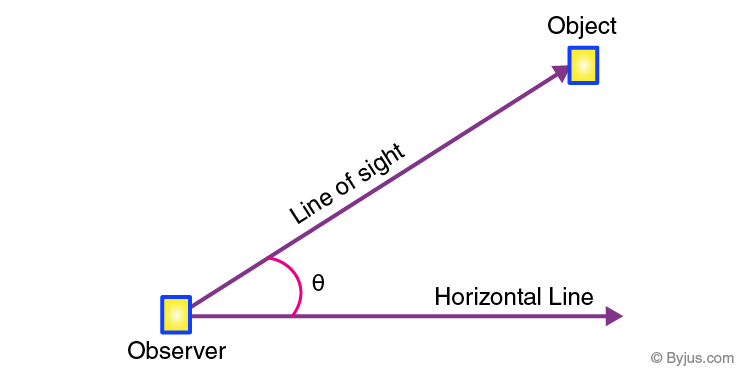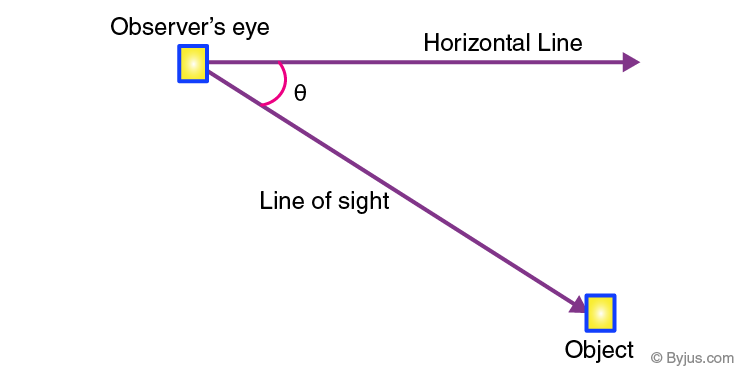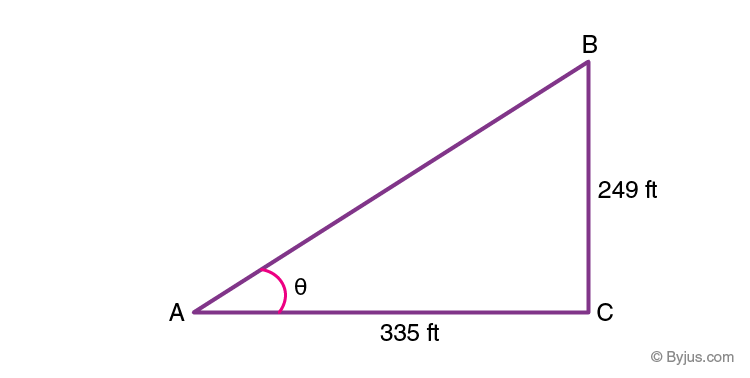Checkout JEE MAINS 2022 Question Paper Analysis : Checkout JEE MAINS 2022 Question Paper Analysis :

# Angle of Elevation

The angle of elevation is a widely used concept related to height and distance, especially in trigonometry. It is defined as an angle between the horizontal plane and oblique line from the observer’s eye to some object above his eye. Eventually, this angle is formed above the surface. As the name itself suggests, the angle of elevation is so formed that it is above the observer’s eye.

For example, an observer is looking at a bird sitting at the rooftop, then there is an angle formed, which is inclined towards the bird from the observer’s eye. This elevation angle is used in finding distances, heights of buildings or towers, etc with the help of trigonometric ratios, such as sine, cosine and tangent.

## Angle of Elevation Definition

The angle of elevation is an angle that is formed between the horizontal line and the line of sight. If the line of sight is upward from the horizontal line, then the angle formed is an angle of elevation.In the above figure, you can see, an observer is looking at the object, standing on the ground, forming an angle θ with the line of sight and horizontal line. Here, if we join an imaginary line between the object and end of the horizontal line, a right angle triangle is formed. Thus we can use here trigonometry concept to find the distance of the observer from the tower or building. Height of the tower or building or the height at which the object is kept will be considered as perpendicular and the horizontal line will be considered as adjacent side of the triangle formed. The related terminology are given here.

## Terms Used for Angle of Elevation

The three terms related to the angle of elevation are

• Angle
• Horizontal Line
• Line of sight

### Angle

If two rays or two line segments meet at a common endpoint, then the point is known as the vertex. Two straight lines meet at a common point is said to form an angle. Angle play an important role, and some other definitions of angle are:

• The angle is a gap in between two lines which connect on one side.
• The angles are measured in degrees.

### Horizontal Line

A straight line on the coordinate flat surface where all points on the line have the same y-coordinate. The angle and horizontal line combine to form the angle of elevation.

### Line of Sight

The line which is drawn from the eyes of the observer to the point being viewed on the object is known as the line of sight.

Here, the object is kept above the line of sight of the observer. If we know the elevation angle, then we can easily determine the distance or the altitude. The reason why we use here trigonometric functions is that the angle formed with the respect to the observer’s eye to the top of a building or a tower produces an imaginary right triangle, where the height of the building or tower is considered as the perpendicular of the triangle.

## Angle of Elevation Formula

The formula for finding the angle of elevation depends on knowing the information such as the measures of the opposite, hypotenuse, and adjacent side to the right angle. If the distance from the object and height of the object is given, then the formula for the angle of elevation is given by

Tangent of the angle of elevation = Height of the Object / Distance from the object

or

Tan θ = Opposite Side/Adjacent Side

## Angle of Elevation and Depression Comparison

The angle of depression is just the opposite scenario of the angle of elevation. In this case, the observer is standing at height and the object is kept below the line of sight of the observer. We can define it as if the object is kept below the eye level of the observer, then the angles formed between the horizontal line and the observer’s line of sight is called the angle of depression.The formula of the angle formed here is given by;

tangent of angle of depression = Opposite side/adjacent side

## Angle of Elevation Examples

Question 1: Find the value of θ in the given figure.Solution:

Given:

In the given triangle ABC, AC = 335 ft, BC = 249 ft

To find ∠A = θ

tan θ = Opposite side/ Adjacent side

tan θ = BC / AC

tan θ = 249/ 335

tan θ = 0.74

Therefore, θ = tan-1 (0.74) = 36

Hence, the value of θ = 36 degrees.

Question 2: If the angle of inclination formed by an observer to an object, kept above a tower, is 45 degrees. Find the horizontal distance between the observer and the height of the tower is 150 ft.

Solution: Given, θ = 45

Height of tower = 150 ft.

To find: the distance between the observer and base of the tower.

By the formula, we know,

tan θ  = Height of the tower/Distance between observer and tower

tan 45 = 150/D

since tan 45 = 1

Therefore,

1 = 150/D

D = 150 ft.

Hence, the distance between the observer and tower is equal to the height of the tower.

To learn more Maths-related articles, stay tuned with BYJU’S – The Learning App and also watch engaging videos to learn with ease.